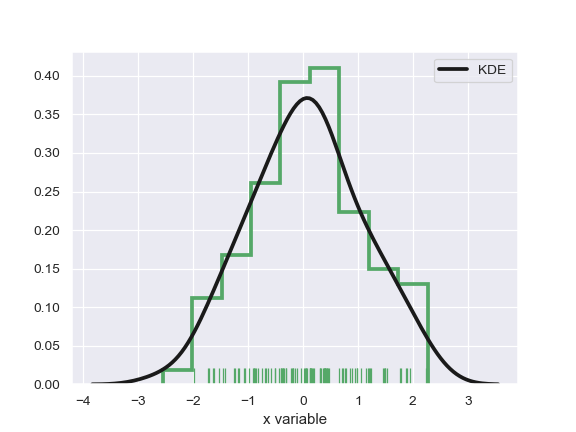# seaborn.distplot¶

`seaborn.``distplot`(a, bins=None, hist=True, kde=True, rug=False, fit=None, hist_kws=None, kde_kws=None, rug_kws=None, fit_kws=None, color=None, vertical=False, norm_hist=False, axlabel=None, label=None, ax=None)

Flexibly plot a univariate distribution of observations.

This function combines the matplotlib `hist` function (with automatic calculation of a good default bin size) with the seaborn `kdeplot()` and `rugplot()` functions. It can also fit `scipy.stats` distributions and plot the estimated PDF over the data.

Parameters
aSeries, 1d-array, or list.

Observed data. If this is a Series object with a `name` attribute, the name will be used to label the data axis.

binsargument for matplotlib hist(), or None, optional

Specification of hist bins. If unspecified, as reference rule is used that tries to find a useful default.

histbool, optional

Whether to plot a (normed) histogram.

kdebool, optional

Whether to plot a gaussian kernel density estimate.

rugbool, optional

Whether to draw a rugplot on the support axis.

fitrandom variable object, optional

An object with fit method, returning a tuple that can be passed to a pdf method a positional arguments following a grid of values to evaluate the pdf on.

hist_kwsdict, optional

Keyword arguments for `matplotlib.axes.Axes.hist()`.

kde_kwsdict, optional

Keyword arguments for `kdeplot()`.

rug_kwsdict, optional

Keyword arguments for `rugplot()`.

colormatplotlib color, optional

Color to plot everything but the fitted curve in.

verticalbool, optional

If True, observed values are on y-axis.

norm_histbool, optional

If True, the histogram height shows a density rather than a count. This is implied if a KDE or fitted density is plotted.

axlabelstring, False, or None, optional

Name for the support axis label. If None, will try to get it from a.name if False, do not set a label.

labelstring, optional

Legend label for the relevant component of the plot.

axmatplotlib axis, optional

If provided, plot on this axis.

Returns
axmatplotlib Axes

Returns the Axes object with the plot for further tweaking.

`kdeplot`

Show a univariate or bivariate distribution with a kernel density estimate.

`rugplot`

Draw small vertical lines to show each observation in a distribution.

Examples

Show a default plot with a kernel density estimate and histogram with bin size determined automatically with a reference rule:

```>>> import seaborn as sns, numpy as np
>>> sns.set(); np.random.seed(0)
>>> x = np.random.randn(100)
>>> ax = sns.distplot(x)
```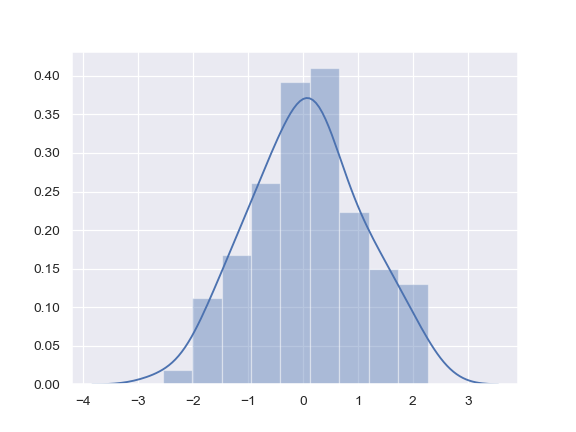Use Pandas objects to get an informative axis label:

```>>> import pandas as pd
>>> x = pd.Series(x, name="x variable")
>>> ax = sns.distplot(x)
```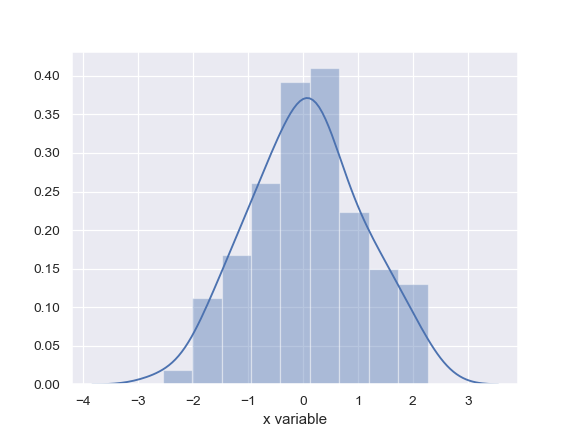Plot the distribution with a kernel density estimate and rug plot:

```>>> ax = sns.distplot(x, rug=True, hist=False)
```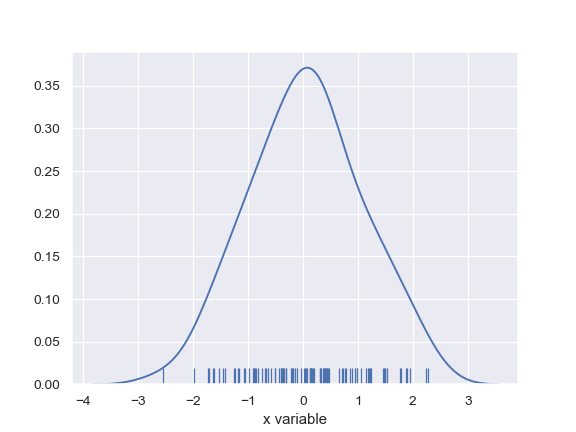Plot the distribution with a histogram and maximum likelihood gaussian distribution fit:

```>>> from scipy.stats import norm
>>> ax = sns.distplot(x, fit=norm, kde=False)
```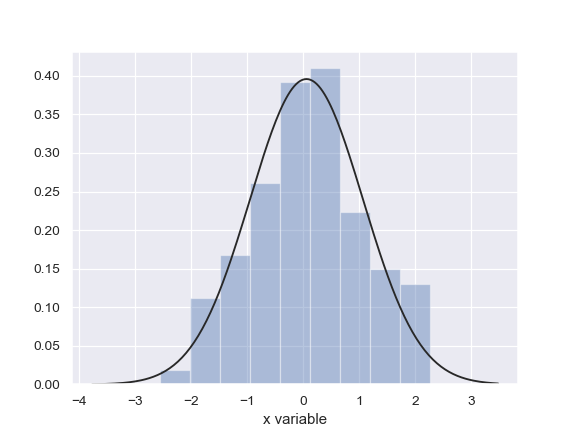Plot the distribution on the vertical axis:

```>>> ax = sns.distplot(x, vertical=True)
```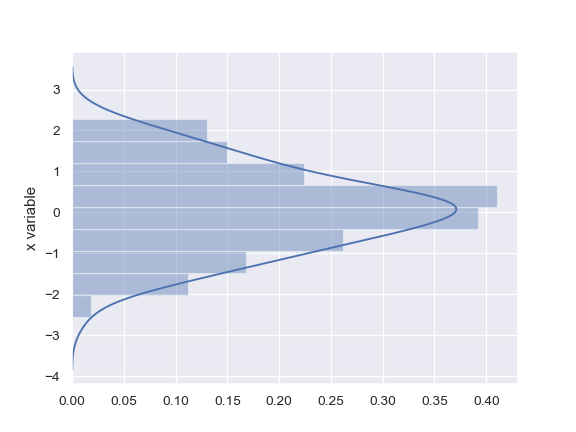Change the color of all the plot elements:

```>>> sns.set_color_codes()
>>> ax = sns.distplot(x, color="y")
```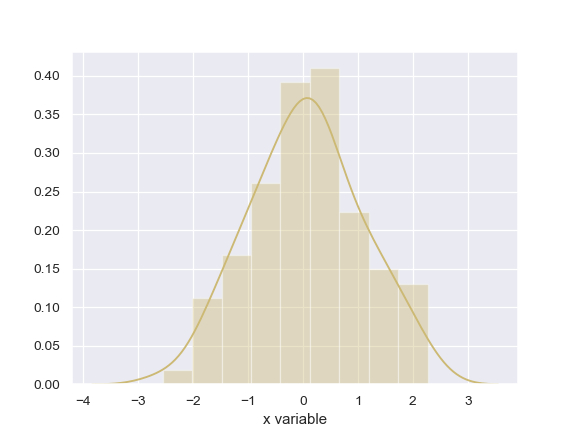Pass specific parameters to the underlying plot functions:

```>>> ax = sns.distplot(x, rug=True, rug_kws={"color": "g"},
...                   kde_kws={"color": "k", "lw": 3, "label": "KDE"},
...                   hist_kws={"histtype": "step", "linewidth": 3,
...                             "alpha": 1, "color": "g"})
```